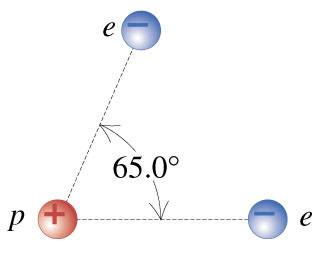# Electrical Force

merlos
A. If two electrons are each 1.60×10−10 m from a proton, as shown in the figure, find the magnitude and of the net electrical force they will exert on the proton.B. Find the direction of the net electrical force electrons will exert on the proton.
Enter your answer as an angle measured relative to the line connecting the proton and the right electron with counterclockwise being positive.

Using the Law of Cosines, I was able to find the angles to be 57.46 and 57.54 degrees.
Csquared = Asquared + Bsquared - 2ABcos(65degrees)
C= 1.72*10^-10

Then I used C/sin(thetac) = A/sin(thetaa)
(1.72*10^-10)/sin(65degrees) = (1.6*10^-10)/sina
a = 57.46 degrees
b = 57.54 degrees

Then I did vector addition to find Fnet. The x-component = 1.54*10^-8 and the y-component = -4.84*10^-9

Therefore Fnet = 1.054*10^-8

??? Am I on the right track??

## The Attempt at a Solution

Mentor
Doesn't look right to me. Are the electrons going to attract or repel the proton? What is the equation for the force exerted by one charge on another in terms of the charges and the distance?

Then the vector addition has nothing to do with the law of cosines. The force from the right electron on the proton will be straight horizontal (which way?), and the force from the other electron will be in line with that 65 degree line. You add the x and y components to get the net.

*best&sweetest*
You do NOT need to calculate any angles. Everything you need is there.
Are you familiar with Coulomb's law and vector addition?
You should know that electrons will attract proton with forces along the lines that connect them - therefore you know the angles.

Mentor
Here's a hint: You should be able to find the direction of the net force on the proton with no calculation whatsoever. Think symmetry. (Of course, you'll still need Coulomb's law to find the magnitude of that force.)